# Principles of lock-in detection and the state of the art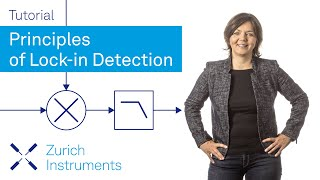## Introduction

Lock-in amplifiers were invented in the 1930's  and commercialized  in the mid 20th century as electrical instruments capable of extracting signal amplitudes and phases in extremely noisy environments (see Figure 1). They employ a homodyne detection scheme and low-pass filtering to measure a signal's amplitude and phase relative to a periodic reference. A lock-in measurement extracts signals in a defined frequency band around the reference frequency, efficiently rejecting all other frequency components. The best instruments on the market today have a dynamic reserve of 120 dB , which means they are capable of accurately measuring a signal in the presence of noise up to a million times higher in amplitude than the signal of interest.

Over decades of development, researchers have found many different ways to use lock-in amplifiers. Most prominently they are used as precision AC voltage and AC phase meters, noise measurement units, impedance spectroscopes, network analyzers, spectrum analyzers and phase detectors in phase-locked loops. The fields of research comprise almost every length scale and temperature, such as the observation of the corona in full sunlight , measuring the fractional quantum Hall effect , or direct imaging of the bond characteristics between atoms in a molecule . Lock-in amplifiers are extremely versatile. As essential as spectrum analyzers and oscilloscopes, they are workhorses in all kinds of laboratory setups, from physics to engineering and life sciences. As with most powerful tools, only a solid understanding of the working principles and features enables the user to get the most out of it and to successfully design experiments.

This document provides a quick introduction to the principles of lock-in amplification and explains the most important measurement settings. The lock-in detection technique is described both in the time and in the frequency domain. Moreover, details are laid out on how signal modulation can be exploited in order to improve on signal-to-noise ratio (SNR) while keeping acquisition time low. Finally, recent innovations are discussed and the state of the art is described.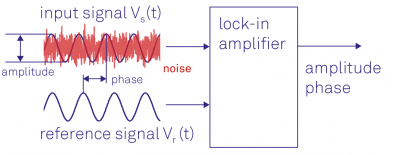Figure 1: Lock-in amplifiers are capable of measuring the amplitude and the phase of a signal relative to a defined reference signal, even if the signal is entirely buried in noise.

## Lock-in amplifier working principle

Lock-in amplifiers use the knowledge about a signal's time dependence to extract it from a noisy background. A lock-in amplifier performs a multiplication of its input with a reference signal, also sometimes called down-mixing or heterodyne/homodyne detection, and then applies an adjustable low-pass filter to the result. This method is termed demodulation or phase-sensitive detection and isolates the signal at the frequency of interest from all other frequency components. The reference signal is either generated by the lock-in amplifier itself or provided to the lock-in amplifier and the experiment by an external source.

The reference signal is usually a sine wave but could have other forms, too. Demodulation with a pure sine wave enables selective measurement at the fundamental frequency or any of its harmonics. Some instruments use a square wave  which also captures all odd harmonics of the signal and, therefore, potentially introducing systematic measurement errors.

To understand lock-in detection, we will look at both the time and the frequency domain, first for mixing and then for the filtering process.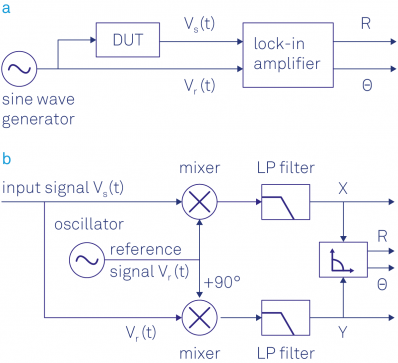Figure 2: (a) Sketch of a typical lock-in measurement. A sinusoidal signal drives the DUT and serves as a reference signal. The response of the DUT is analyzed by the lock-in which outputs the amplitude and phase of the signal relative to the reference signal. (b) Schematic of the lock-in amplification: the input signal is multiplied by the reference signal and a 90° phase-shifted version of the reference signal. The mixer outputs are low-pass filtered to reject the noise and the 2ω component, and finally converted into polar coordinates.

### Dual-phase demodulation

In a typical experiment, the device under test (DUT) is stimulated by a sinusoidal signal, as shown in Figure 2 (a). The device response Vs(t) as well as the reference signal Vr(t) are used by the lock-in amplifier to determine the amplitude R and phase Θ. This is achieved using a so-called dual-phase demodulation circuit, as illustrated in Figure 2 (b). The input signal is split and separately multiplied with the reference signal and a 90° phase-shifted copy of it. The outputs of the mixers pass through configurable low-pass filters, resulting in the two outputs X and Y, termed the in-phase and quadrature component. The amplitude R and the phase Θ are easily derived from X and Y by a transformation from Cartesian coordinates into polar coordinates using the relation

\begin{align*} &R=\sqrt{X^2+Y^2},\\ &\Theta = atan2(Y,X) \end{align*}
(1)

Note that in order to have an output range for the phase angle that covers all four quadrants, i.e. (-π, π], atan2 is used instead of atan.

Figure 2 (b) shows that the lock-in amplifier has to split up the input signal in order to demodulate it with two different phases. Contrary to analog instruments, digital technology overcomes any losses in SNR and mismatch between the channels when splitting the signal.

### Signal mixing in the time domain

Complex numbers provide an elegant mathematical formalism to calculate the demodulation process. We use the elementary trigonometric law

$cos(x)=\frac{1}{2}e^{+ix}+\frac{1}{2}e^{-ix}$
(2)

to rewrite the input signal Vs(t) as the sum of two vectors in the complex plane, each one of length R/√2 rotating at the same speed ωs, one clockwise and the other counter-clockwise:

\begin{align*} V_S(t)&=\sqrt{2}R\cdot cos(\omega_St+\Theta) \\ &= \frac{R}{\sqrt{2}}e^{+i(\omega_st+\Theta)}+\frac{R}{\sqrt{2}}e^{-i(\omega_st+\Theta)}. \end{align*}
(3)

In the graphical representation given in Figure 3 (a) and (b) one can see that the vectors' sum projected on the x-axis – the real part – is exactly Vs(t), whereas the vector sum projection onto the y-axis – the imaginary part – is always zero.

The dual-phase down-mixing is mathematically expressed as a multiplication of the input signal with the complex reference signal

$V_r(t)=\sqrt{2}e^{-i\omega_rt}=\sqrt{2}cos(\omega_rt)-i\sqrt{2}sin(\omega_rt).$
(4)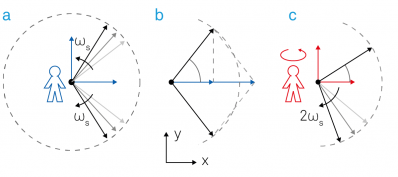Figure 3: Demodulation process represented in the complex plane. (a) The input signal Vs(t) can be expressed as the sum of two counter-rotating vectors. (b) The projections onto the x-axis add up whereas the projections to the imaginary y-axis cancel each other out. (c) In the rotating frame the counter-clockwise vector is standing still, the clockwise moving vector rotates at twice the observer's angular velocity. Note that by convention, Θ is positive if the counter-clockwise vector is ahead of the reference.

The complex signal after mixing is given by

\begin{align*} Z(t) &= X(t)+iY(t)=V_S(t)\cdot V_r(t)\\ &= R\left [ e^{i\left [ (\omega_s-\omega_r)t+\Theta \right ]} +e^{-i\left [ (\omega_s+\omega_r)t+\Theta \right ]}\right ], \end{align*}
(5)

with signal components at the sum and the difference of the signal frequency and the reference frequency. In the picture of Figure 3 (c), the complex mixing is equivalent to an observer located at the origin and rotating in a counter-clockwise direction with frequency ωr.

In the eyes of this observer, the two arrows appear to rotate at different angular velocities ωsr and ωs + ωr, with the arrow ωs + ωr rotating much faster if the signal and reference frequencies are close.

The subsequent filtering is mathematically expressed as an averaging of the moving vectors over time, indicated by the angle brackets $\left \langle ... \right \rangle$. Filtering strips away the fast rotating term at |ωs + ωr| by setting $\left \langle exp\left [ -i(\omega_s+\omega_r)t+i\Theta \right ] \right \rangle = 0$. The averaged signal after demodulation becomes

$Z(t)=R\cdot e^{i[(\omega_s-\omega_r)t+\Theta]}.$
(6)

In the case of equal frequencies ωs = ωr, this further simplifies to

$Z(t)=R\cdot e^{i\Theta}.$
(7)

Equation 7 is the demodulated signal and the main output of the lock-in amplifier: with the absolute value |Z| = R given as the root-mean-square amplitude of the signal and its argument arg(Z) = Θ given by the phase of the input signal relative to the reference signal.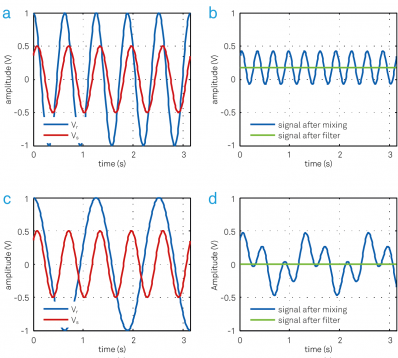Figure 4: (a) An input signal Vs (red) with peak amplitude of 0.5 V is multiplied with the reference signal Vr (blue) at the same frequency. (b) The resulting signal has a DC offset and a frequency component at twice the frequency of Vs and Vr. The DC value is 0.17 V, which is the in-phase component X of the input signal. (c) The input signal Vs is multiplied by a reference Vr at a different frequency. (d) The resulting signal has frequency components at fs - fr and fs + fr. The average signal is always zero.

The real and imaginary parts of the demodulated signal Z(t) are the in-phase component X and the quadrature component Y. They are obtained using Euler's formula exp(iωst) ≡ cos(ωst) + isin(ωst) as:

\begin{align*} X&= Re(Z)=\left \langle V_s(t)cos(\omega_st) \right \rangle=R \; cos \; \Theta,\\ Y&= Im(Z)=-\left \langle V_s(t)sin(\omega_st) \right \rangle=R \; sin \; \Theta. \end{align*}
(8)

In the graphical view, ωs = ωr means that the arrow rotating counter-clockwise will appear at rest. The other arrow is rotating clockwise at twice the frequency, i.e. -2ωs, and is often called the 2ω component. The low-pass filter usually cancels out the 2ω component completely.

Figure 4 illustrates the different signals before and after mixing and filtering as they would appear on an oscilloscope. Figure 4 (a) shows the sinusoidal example signals Vs and Vr over time having exactly the same frequencies ωs and ωr. The signal after mixing, blue trace in Figure 4 (b), is dominated by the 2ω component. After filtering, green trace, only the DC component remains, which is equal to the in-phase amplitude X of Vs. If the signal frequency and the reference frequency deviate, as shown in Figure 4 (c), the resulting signal after mixing is no longer a simple sine wave and averages out to zero after filtering, as shown in Figure 4 (d). It is the perfect example of synchronous detection, which exclusively extracts signals coherent with the reference frequency and discards all others.

### Signal mixing in the frequency domain

To switch between the time domain and the frequency domain picture, we use the Fourier transform . The Fourier transform is linear and converts a sinusoidal function with frequency f0 in the time domain into a Dirac delta function δ(f-f0) in the frequency domain, i.e. a single peak at frequency f0 in the spectrum. As any periodic signal can be expressed as a superposition of sines and cosines , transformations of signals consisting of only a few spectral components can often be intuitively understood.

Figure 5 (a) shows a noisy sinusoidal represented in the time domain, which is then Fourier transformed into the frequency domain in Figure 5 (b). The sinusoidal signal shows up as a peak both at +fs and at -fs in the spectrum. The smaller peak at zero frequency is caused by the input signal's DC offset. The blue trace in Figure 5 (c) represents the time domain signal after mixing. The associated spectrum shown in Figure 5 (d) is essentially a copy of the one in (b) shifted by the reference frequency fr towards lower frequencies.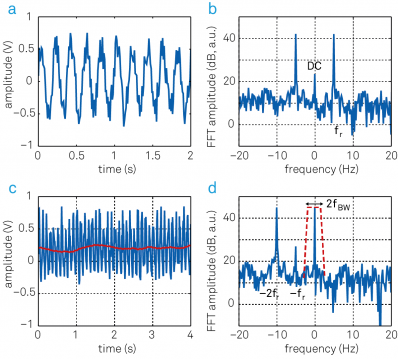Figure 5: Relationship between time and frequency domain representation before and after demodulation. (a) Sinusoidal input signal superimposed with noise displayed over time. (b) Same signal as in (a) represented in the frequency domain. (c) After mixing with the reference signal (blue trace) and low-pass filtering (red trace), the signal spectrum up to fBWremains. (d) In the frequency representation, the frequency-mixing shifts the frequency components by -fr. The filter then picks out a narrow band of fBWaround zero. Note the component at frequency -fs, which comes from offset and 1/f noise in the input signal. To obtain accurate measurements this component has to be suppressed by proper filtering.

Low-pass filtering is indicated as a dashed red trace in (d) and selects the frequencies up to a certain filter bandwidth fBW. The output signal, red trace in (c), is the DC component of the spectrum visualized in (d) plus the noise contribution within the filter bandwidth |f| < fBW. It is evident from this picture that a filter bandwidth significantly smaller than the signal frequency fs is required to efficiently suppress offsets in the input signal. In the next sections, we'll discuss further criteria for choosing suitable filter characteristics in a given experimental situation.

### Low-pass filtering in the frequency domain

For the low-pass filtering we start by considering the frequency domain because for most filters there is a simple relationship between the incoming signal Qin(ω) and the filtered signal Qout(ω) given by

$Q_{out}(\omega)=H(\omega)Q_{in}(\omega).$
(9)

H(ω) is called the transfer function of the filter. Qin(ω) and Qout(ω) are the Fourier transforms of the time domain input signal Qin(t) and output signal Qout(t) respectively.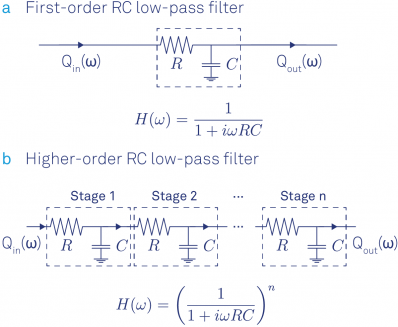Figure 6: (a) First-order RC filter and its transfer function formula. (b) Steeper roll-offs towards higher frequencies are achieved by stacking multiple RC filters. The transfer function results from a multiplication of each filter's transfer function.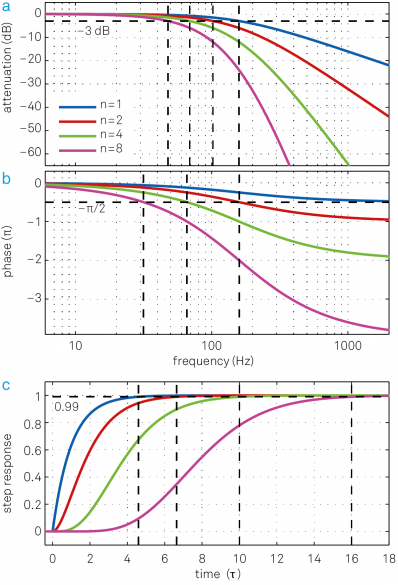Figure 7: The blue traces in (a) and (b) show the transfer function H(ω) of an RC filter in the form of a Bode plot. The transfer functions for higher-order filters (n = 2, 4, 8) with the same filter time constant τ are also plotted and clearly have much lower signal bandwidth f-3dB. (c) Associated step response functions in the time domain. Cascading multiple filters leads to a significant increase in settling time to achieve the same level of accuracy. This is related to the larger phase delay that is inferred from (b). One additional nice feature of the cascaded RC or integrator filter is that it has no overshoot in the time domain, which is an issue with Butterworth filter for instance.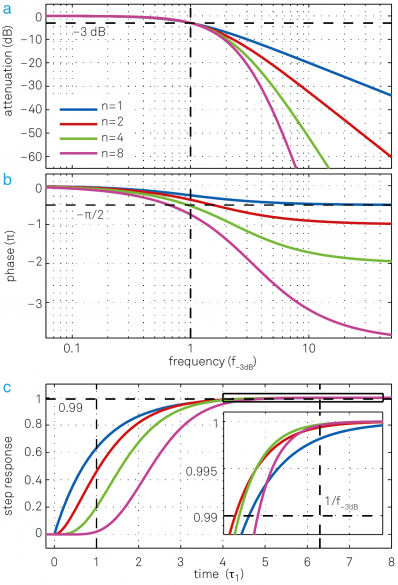Figure 8: Same set of plots as for Figure 7 but this time all filters have the same cut-off point f-3dB but different time constants τ = 0.16, 0.10, 0.069, 0.048. (a) Higher-order filters show a steeper roll-off towards higher frequencies. (b) Higher-order filters have larger phase delays, which can be detrimental for feedback applications. (c) Step response as a function of time in units of the time constant τ 1 of the first-order filter. Though lower-order filters respond more quickly to changes of the input signal at the beginning, this advantage decreases over time and at some point higher-order filters even “overtake” lower-order filters, as seen in the inset.

To perfectly reject unwanted parts of the spectrum, one might think that an ideal filter should have full transmission for all frequencies below fBW, i.e. the passband, and zero transmission for all other frequencies, also called the stop band. Unfortunately such idealized “brick-wall filters” are impossible to realize since their impulse response extends from -∞ to +∞ in time, which makes them non-causal. As a basic approximation, we consider the RC filter model, see Figure 6. This type of filter is easy to implement both in the analog and the digital domain. The transfer function of an analog RC filter is well approximated by

$H(\omega)=\frac{1}{1+i\omega\tau},$
(10)

where τ  = RC is called the filter time constant with the resistance R and capacitance C. The blue traces in Figure 7 (a) and (b) show this transfer function in Bode plots, 20log|H(2πf)| and arg[H(2πf)] as functions of log(f).

 Order Time Roll-off Bandwidth in units of 1/τ Settling times in units of τ n constant τ dB/oct dB/dec f-3dB fNEP fNEP/f-3dB 63.2% 90% 99% 99.9% 1 1 6 20 0.159 0.250 1.57 1.00 2.30 4.61 6.91 2 1 12 40 0.102 0.125 1.23 2.15 3.89 6.64 9.23 3 1 18 60 0.081 0.094 1.16 3.26 5.32 8.41 11.23 4 1 24 80 0.069 0.078 1.13 4.35 6.68 10.05 13.06 5 1 30 100 0.061 0.069 1.12 5.43 7.99 11.60 14.79 6 1 36 120 0.056 0.062 1.11 6.51 9.27 13.11 16.45 7 1 42 140 0.051 0.057 1.11 7.58 10.53 14.57 18.06 8 1 48 160 0.048 0.053 1.10 8.64 11.77 16.00 19.62
Table 1: Overview of the filter properties of nth order RC filters with the same time constant. Dynamic applications usually take into consideration f-3dB and settling times, whereas for noise measurements taking into account the correct fNEP is key to achieve accurate results. With the relations given above one can easily calculate filter time constants for filters of the same bandwidth but different order.

From the blue curve in Figure 7 (a) we can infer that the attenuation grows ten times every tenfold frequency increase above f-3dB. This equals 6 dB/octave (20 dB/decade) corresponding to an amplitude reduction by a factor of 2 every doubling of the frequency. The cut-off frequency f-3dB is defined as the frequency at which the signal power is reduced by -3 dB or one half. The amplitude, proportional to the square root of the power, is reduced by 1/√2 = 0.707 at f-3dB.

For the filter described by Equation 10, the cut-off frequency is f-3dB = 1/(2πτ). From Figure 7 (b) we see that the low-pass filter also introduces a frequency dependent phase delay equal to arg[H(ω)].

Compared to the idealized brick-wall filter, the first-order filter has a fairly poor roll-off behavior. To increase the roll-off steepness it is common to cascade several of these filters. For every filter added the filter order is increased by 1. Since the output of one filter becomes the input to the following one, we can simply multiply their transfer functions. From subsection 9 we thus get the following transfer function of an nth order filter:

$H_n(\omega)=H_1(\omega)^n=(\frac{1}{1+i\omega\tau})^n.$
(11)

Its attenuation is n times the attenuation of a first-order filter, with a total roll-off of n × 20 dB/dec. The frequency responses of a 1st, 2nd, 4th and an 8th order RC filter are shown in Figure 7 (a) and (b). The higher the filter order, the closer the amplitude transfer function gets to a brick-wall filter behavior. At the same time, the phase delay increases with filter order. For applications where the phase is used to apply a feedback to a system, for example phased-locked loops, any additional phase delay can limit the stability and bandwidth of the control loop.

Figure 8 (a) and (b) show the Bode plots for filters of different orders with the same bandwidths f-3dB but different time constants. Table 1 provides the numerical relationship between corresponding filter properties.

For noise measurements, it's often more useful to specify a filter in terms of its noise equivalent power bandwidth fNEP, rather than the 3 dB bandwidth f-3dB. The noise equivalent power bandwidth is the cut-off frequency of an ideal brick-wall filter that transmits the same amount of white noise as the filter we wish to specify. For cascaded RC filters, the conversion factor between fNEP and f-3dB is listed in Table 1.

After mixing the input signal Vs(t) with the reference signal √2 exp(-iωrt), the input signal spectrum is shifted by the demodulation frequency ωr and becomes Vs(ω-ωr). Low-pass filtering further transforms the spectrum through a multiplication by the filter transfer function Hn(ω). The demodulated signal Z(t) contains all frequency components around the reference frequency, weighted by the filter response

$Z(\omega)=V_s(\omega-\omega_r)H_n(\omega).$
(12)

This equation clearly shows that demodulation behaves like a bandpass filter in that it picks out the frequency spectrum centered at fr and extending on each side by f-3dB. Moreover, it shows that one can recover the spectrum of the input signal around the demodulation frequency fr by dividing the Fourier transform of the demodulated signal by the filter transfer function. This form of spectral analysis is often used by FFT spectrum analyzers and sometimes referred to as zoomFFT .

### Low-pass filter in the time domain

The time domain characteristics of a filter is best visualized by its step response, as shown in Figure 7 (c) and Figure 8 (c). These plots correspond to a situation where the input of the filter is changed in a step-like fashion from 0 to 1. A certain amount of time will be needed before the filter output settles at the new value. In order to measure a signal that has passed through a filter accurately, the experimentalist must wait for a settling time long enough before taking the measurement.

Table 1 lists the times to reach 63.2%, 90%, 99% and 99.9% of the final value for filters of different orders but identical time constant τ . Assume we have a 1 MHz signal and want to use a 4th-order filter with a bandwidth of 1 kHz around 1 MHz. From the numbers given in Table 1 we can derive that the time constant is 69 µs and the settling time to 1% error is 0.7 ms.

## Signal dynamics and demodulation bandwidth

Setting the demodulation bandwidth is often a tradeoff between time resolution and SNR. Let's consider an amplitude modulated (AM) input signal with carrier frequency fc = ωc/2π,

$V_s(t)=\left [ 1+h\, cos(\omega_mt) \right ]\,cos(\omega_ct+\varphi_c)$
(13)

represented in Figure 9 as an example to discuss how requirements for different experimental questions can be met. The signal amplitude R(t) = 1 + hcos(ωmt), the blue trace in the Figure 9, is modulated at a frequency fm = ωm/2π around the average value 1, where the modulation index h characterizes the modulation strength. For this example we choose carrier and modulation frequencies of fc = 2 kHz and fm = 100 Hz, respectively.

Using the complex representation introduced with Figure 3, Figure 10 (a) shows the AM signal after mixing. Its modulus |1 + hcos(ωmt)| is time-dependent but its angle φc is constant. The term cos(ωmt) is the sum of the two counter-rotating vectors exp(iωmt) and exp(-iωmt). These two vectors represent the upper and lower sidebands of the frequency spectrum of an amplitude modulated signal, as seen in Figure 10 (d). Figure 10 (b) and (c) show the quadrature and in-phase component, respectively.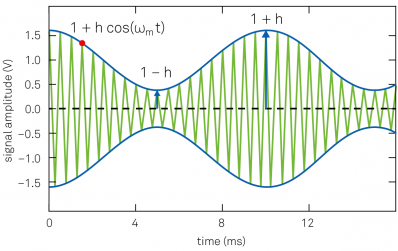Figure 9: Amplitude modulated signal: the green trace is the carrier input signal (displayed at a lower frequency for clarity). The blue trace indicates the signal amplitude, which is the envelope of the input signal.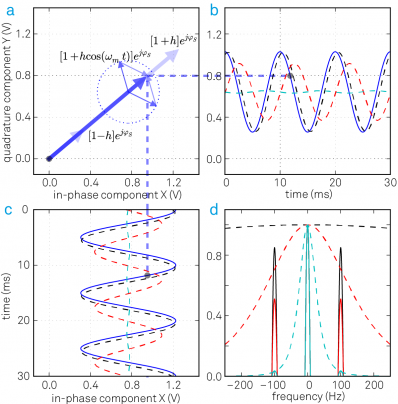Figure 10: (a) An amplitude modulated signal in the rotating frame of reference is a vector with a time dependent length. The instantaneous signal is represented by the thick blue arrow; the thinner arrows display the two sidebands of the AM signal. (b) and (c) the quadrature and in-phase components of the demodulated input signal: the blue trace is the unfiltered signal, the dashed black, red and cyan traces are the filtered signals with f-3dB = 500 Hz, 100 Hz and 20 Hz, respectively. (d) The frequency spectrum of the demodulated signal after filtering with three different bandwidths (black, red and cyan curves).

Most applications require measuring one of the following quantities:

• the time dependence of the amplitude R(t) = 1 + hcos(ωmt)
• the average value of the amplitude ⟨R(t)⟩
• the modulation index h

In the first situation, we would like the demodulated signal to follow amplitude changes at a rate fm. This requires a filter bandwidth significantly larger than fm. Consider for instance a 4th-order filter with a bandwidth of f-3dB = 500 Hz. With this choice, the transmission at fm = 100 Hz (that is 100 Hz away from the carrier fc) is about 98.5% and the phase delay is about 20° as one can calculate from Equation 11 and Table 1. In other words, the modulation signal is only slightly affected by the filter. The demodulated signal is displayed as the dashed black line in Figure 10 (b) and (c).

Apart from the desired sideband suppression/admission and phase delay, the amount of noise in the measurement is an important criterion in the choice of a filter. Figure 11 illustrates this with an AM signal with relatively strong noise after demodulation in (a). Panel (b) shows the same signal after filtering with a cutoff frequency equal to the modulation frequency. While this filter eliminates most of the noise, it introduces systematic changes in the amplitude and phase that need to be corrected to get accurate results.

For the second set of requirements, frequency components corresponding to the sidebands are rejected by reducing the filter bandwidth to a value smaller than fm. A 4th-order filter with f-3dB = 20 Hz, dashed cyan line in Figure 10 (d), suppresses the sidebands by 0.03 or 30 dB. Figure 11 (c) illustrates the effect of such a strong filter on the measurement.

In the third case, we want to know the modulation index h but don't need to resolve the full signal dynamics. This is used, for instance, in Kelvin probe force microscopy, where h is a measure of the electrostatic force between a probe and a sample in response to an alternating voltage at fm. Since the modulation index is proportional to the amplitude of the sidebands, this measurement can be performed by applying narrow filters around the sidebands at fc-fm and fc+fm. There are two ways to do this: by so-called tandem demodulation or by direct sidebanddemodulation.

In tandem demodulation, we first perform a wide-band demodulation around the center frequency. The resulting signal, typically looks similar to the one in Figure 11 (a), is then demodulated again at fm. The modulation frequency accessible with this method can't be larger than the maximum demodulation bandwidth of the first lock-in unit. In direct sideband demodulation, the signal is demodulated at fc ± fm in a single step, and the accessible modulation frequencies are only limited by the frequency range of the lock-in amplifier. Also, direct sideband demodulation works with a single lock-in amplifier instead of two and is therefore usually the preferred choice.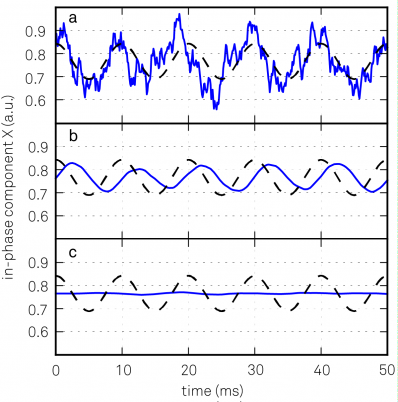Figure 11: (a) A noisy input signal will produce a noisy demodulated signal, blue trace. The underlying signal without the noise is plotted as a black dashed trace. (b) Applying a filter with bandwidth f-3dB = fm = 100 Hz will eliminate most of the noise but will also affect the detected signal. (c) Same as (b) but with f-3dB = fm/5 = 20 Hz.

## Achieving high SNR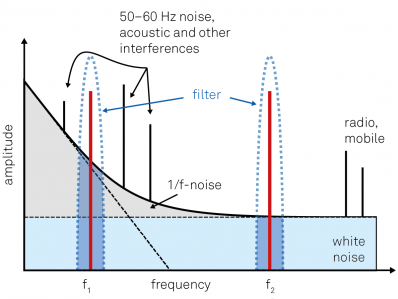Figure 12: Qualitative noise spectrum of a typical experiment. The measurement frequency should be chosen in a region with small background, avoiding any discrete peaks coming from technical sources. In the example, f2 will yield better results than f2 for the same filter bandwidth, since it is located in a clean white noise region above the 1/f noise at low frequencies.

Reducing the filter bandwidth generally leads to higher SNR at the expense of time resolution. What other measures can be taken to improve SNR?

If the signal strength cannot be increased, the noise has to be reduced or avoided as much as possible. However, noise is always present in analog signals and arises from different sources, some of which are of fundamental origin, for example Johnson-Nyquist (thermal) noise, shot noise and flicker noise, while others are of technical origin, as for example ground loops, interference, cross-talk, 50–60 Hz noise or electromagnetic pick-up. The magnitude of a random voltage noise Vnoise(t) is specified by its standard deviation. In the frequency domain, noise is characterized by its power spectral density |vn(ω)|2 in units of V2/Hz, or by |vn(ω)| in units of V/√Hz.

The qualitative spectrum in Figure 12 shows that different noise sources have different frequency dependencies: while Johnson-Nyquist noise has a flat spectrum for all practical frequencies and therefore contributes to the “white noise”, flicker noise has a 1/f frequency dependence (“pink noise”). If there is some freedom in the choice of modulation frequency, we can zoom in to a part of the spectrum where the noise level is lowest. Often higher frequencies where the spectrum consists of white noise characteristics work best. Figure 12 illustrates this approach: the amount of noise inside a filter, indicated by the blue and gray filled area, is larger for example in the lower frequency 1/f noise region. Hence, the SNR at f2 is higher than at f1 using the same filter bandwidth, because the noise density is lower as long as other noise sources, such as as radio and wireless transmission are avoided.

To give a more quantitative example, let us assume we want to measure a sinusoidal signal with amplitude of 1 µV across a 1 MΩ resistor with a SNR larger than 10. Such a resistor R exhibits a thermal noise with a power spectral density of $\overline{v_{n}^{2}}$ = 4kBTR, which amounts to about $\sqrt{\overline{v_{n}^{2}}}$ = 0.127 √R nV/√Hz =127 nV/√Hz at T = 300 K room temperature1 . In this example, thermal noise is identified as the dominant noise source. It is clearly stronger than the lock-in input noise of typically less than 10 nV/√Hz. We can thus calculate the SNR as

$SNR=\frac{1\mu V}{127nV/\sqrt{Hz}\cdot\sqrt{f_{NEP}}}=10$
(14)

By solving this equation for fNEP, we calculate that we need to select a NEP filter bandwidth of 620 mHz or less to achieve a SNR of 10. We choose a 4th order filter. From Table 1 we can calculate the corresponding cutoff frequency f-3dB = 549 mHz, the time constant τ = 126 ms, and the settling time to 1% is 1.26 s.

To further increase the SNR by a factor of 10, we would need to decrease the filter bandwidth by a factor of 100, because the noise amplitude is proportional to the square root of the bandwidth. The settling time to 1% then increases to more than 2 minutes. The lock-in technique can support such long measurements because it is insensitive to DC offset drift in the input signal. Nonetheless, other sources of drift such as changes in the DUT resistance, or in amplifier gain, may affect long measurements. Maintaining stable conditions and especially constant temperature are then crucial.

## State of the art

Since the early 1930s lock-in amplifiers have come a long way. Starting from vacuum tubes as basic instrument technology, we note the transition to digital is well underway but not yet complete. In digital lock-in amplifiers, the input signal is immediately converted to the digital domain by an analog-to-digital converter (ADC) and all subsequent steps are then carried out numerically by digital signal processing (DSP), as shown in Figure 13 (b). In contrast, analog lock-in amplifiers use analog elements like voltage-controlled oscillators, mixers and simple RC filters for signal processing. There are also hybrid versions , as sketched in Figure 13 (a), which digitize the signals only after the analog mixing stage before or after filtering.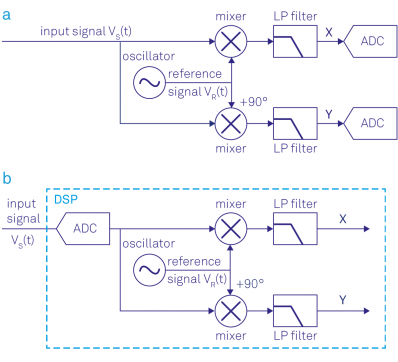Figure 13: (a) Analog lock-in amplifier: the signal is split into two paths, mixed with the reference signal, filtered and then converted to digital. (b) Digital lock-in amplifier: the signal is digitized and then multiplied with the reference signal and filtered.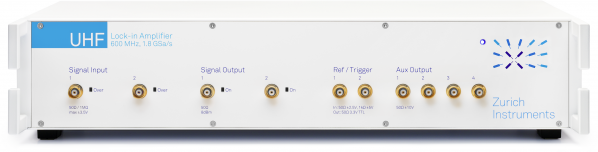Figure 14: Zurich Instruments UHFLI Lock-in amplifier representing the state of the art of lock-in technology. The 600 MHz signal input bandwidth as well as the 5 MHz demodulation bandwidth make it by far the fastest lock-in amplifier on the market today. In addition, the 19 inch wide instrument integrates the greatest amount of functionality, see Figure 16, while providing the most advanced instrument control software LabOne® (see Figure 15).

The transition from analog to digital was fueled by the availability of ADCs and DACs with ever increasing speed, resolution and linearity. This development helped to push the frequency range, input noise and dynamic reserve to new limits. In addition, digital signal processing is much less prone to errors introduced by a mismatch of signal pathways, to cross-talk and to drifts, caused for instance by temperature changes. This is particularly critical at higher frequencies. But the biggest advantage of the digital approach is probably the ability to analyze the signal in multiple ways simultaneously without loss of SNR. As mentioned earlier, this enables not only better dual-phase demodulation, but also the analysis of several frequency components of a signal directly, without the need to cascade multiple instruments with all the accompanying detrimental effects.

After the transition from analog to digital, another significant step of innovation was sparked by the availability of field programmable gate arrays (FPGA) with high computing power, abundant memory and speed. FPGAs are well understood as digital clockworks that can be flexibly programmed to carry out almost any desired signal processing task in real time. The natural extension of the lock-in is to add time domain and frequency domain analysis before and after demodulation, something that would otherwise be done with a separate scope and spectrum analyzer. Furthermore, a single instrument can contain boxcar averagers to analyze signals with low duty cycle, PID and PLL controllers for feedback loops and arithmetic units to process measurement data in real time. The measurement signals can then be transferred to a computer for further analysis. If an analog interface to another instrument is needed, measurement data from different functional units are easily converted back to the analog domain using high-resolution DACs.

The most advanced instrument today when it comes to speed and level of integration is Zurich Instruments' UHFLI , introduced in 2013. Figure 14 shows the front panel of the instrument. The UHFLI has a signal input bandwidth of 600 MHz and a maximum demodulation bandwidth of 5 MHz, which makes it by far the fastest lock-in amplifier on the market today. Despite high speed, it still provides exceptional input noise performance of only 4 nV/√Hz and a dynamic reserve of 100 dB. The high level of integration is illustrated in Figure 16 showing the main functional components of the UHFLI and their interconnections. Functionality that used to require an entire rack of instruments is now housed in a single instrument no larger than a shoe box.

Clearly, the wealth of functionality indicated in Figure 16 cannot be controlled and utilized with a few knobs and buttons on the front panel. Instead, the UHFLI is entirely controlled from a computer running LabOne®, an instrument control software using the latest browser technology that provides a graphical user interface to any device with a web browser, see Figure 15. High-level tools such as the Parametric Sweeper, the Software Trigger, or the PID Advisor, exploit the available processing power of the host computer for measurement tasks, which improves confidence in the measurement results, and enables a more efficient workflow. In addition LabOne also offers programming interfaces for LabVIEW®, MATLAB®, Python and C# to conveniently integrate the measurement instrument into existing experiment control environments.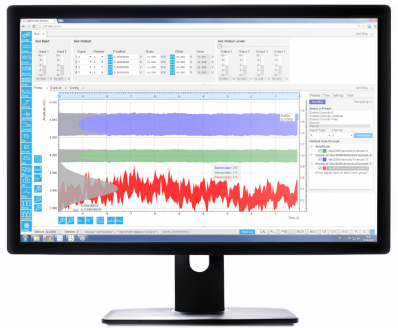Figure 15: The LabOne® user interface of the UHFLI Lock-in amplifier uses the latest web browser technology. The instrument can be controlled from multiple browser sessions on multiple PCs, tablets, etc. at the same time. Every signal analysis and control tool has a dedicated tab. Some of the functionality is intuitively displayed in form of block diagrams.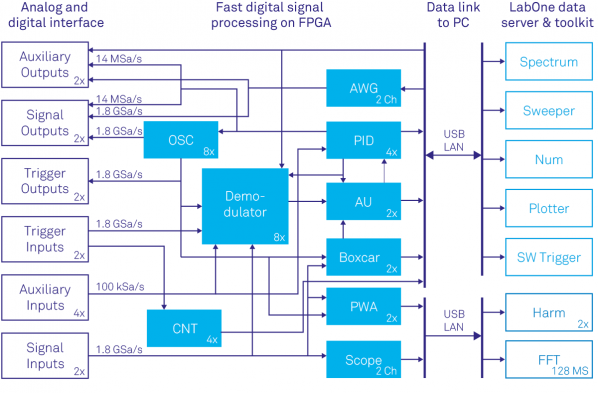Figure 16: Block diagram showing the Zurich Instruments UHFLI's main functional entities and the signal flow between them. Fast digital signal processing takes place inside the instrument's FPGA clocked at 450 MHz but also on the computer connected by USB or 1GbE running the instrument control software LabOne®. The main functional components inside the instrument are the 8 dual-phase demodulators, an oscilloscope (Scope) with digitizer functionality (DIG) and FFT , PID modules with PLL capability, an arithmetic unit (AU), a boxcar averager with periodic waveform analyzer (PWA) and a pulse counter module (CNT). For signal generation the instrument provides sinusoidal signal generators (OSC) and arbitrary waveform generators (AWG) for complex signal shapes. The LabOne control software running on the PC adds a parametric sweeper, a spectrum analyzer, a numerical parameter display (Num), a plotter, a software trigger for time domain analysis and a harmonic analyzer (Harm).
C. R. Cosens. A balance-detector for alternating-current bridges. Proceedings of the Physical Society, 46:818, 1934.
W. C. Michels. A Double Tube Vacuum Tube Voltmeter. Rev. Sci. Instrum., 9:10, 1938.
W. C. Michels and N. L. Curtis. A Pentode LockIn Amplifier of High Frequency Selectivity. Rev. Sci. Instrum., 12:444, 1941.
Interview of Robert Dicke by Martin Hawrit.Niels Bohr Library and Archives, College Park, MD: American Institute of Physics, www.aip.org/history-programs/niels-bohr-library/oral-histories/4572, 1985. Accessed: 2016-10-21.
Zurich Instruments HF2LI. http://www.zhinst.com/products/hf2li. Accessed: 2016-10-21.
A. M. Skellett. The Coronaviser, an Instrument for Observing the Solar Corona in Full Sunlight. Proc Natl Acad Sci USA, 26(6):430, 1940.
D. C. Tsui, H. L. Stormer, and A. C. Gossard. Two-dimensional magnetotransport in the extreme quantum limit. Phys. Rev. Lett., 48:1559, 1982.
L. Gross et al. Bond-Order Discrimination by Atomic Force Microscopy. Science, 337(6100):1326, 2012.
Stanford Research SR844. http://www.thinksrs.com/products/SR844.htm. Accessed: 2016-10-21.
Wikipedia Article: Fourier Transform. https://en.wikipedia.org/wiki/Fourier_transform. Accessed: 2016-10-21.
Wikipedia Article: Fourier Series. https://en.wikipedia.org/wiki/Fourier_series. Accessed: 2016-10-21.
N. Thrane. Zoom-FFT. Brüel & Kjær Technical Review, (2):3, 1980.
Zurich Instruments UHFLI. http://www.zhinst.com/products/uhfli. Accessed: 2016-10-21.